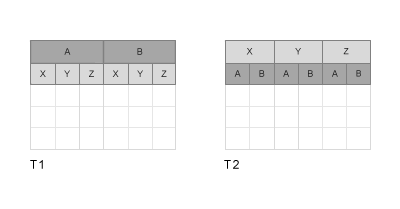# inner2outer

Invert nested table-in-table hierarchy in tables or timetables

## Syntax

``T2 = inner2outer(T1)``

## Description

example

````T2 = inner2outer(T1)` finds the variables in `T1` that are themselves tables or timetables. It returns `T2`, a table or timetable that also contains nested tables or timetables as variables. The names of the variables in `T2` are taken from the names of the variables inside the nested tables or timetables of `T1`. Then, `inner2outer` regroups variables in the nested tables or timetables of `T2` appropriately. If `T1` has variables that are not tables or timetables, then those variables are unaltered in `T2`.For example, if `T1` has two variables named `A` and `B`, and they are each tables with variables named `X`, `Y`, and `Z`, then the output table `T2` has three variables. The variables of `T2` are named `X`, `Y`, and `Z`, each being a table with two variables named `A` and `B`. The table variables `T1.A.X` and `T1.B.X` are regrouped into `T2.X.A` and `T2.X.B`. The other table variables from `T1` are regrouped in `T2` following the same pattern.```

## Examples

collapse all

Load and display a timetable, `T1`, that has nested tables containing stock information. The nested tables `AAPL` and `MSFT` are the variables of `T1`. Each nested table has the stock prices at the open and close of trading, and the volume, for a different company.

```load nestedTables T1 ```
```T1 = 3x2 timetable Dates AAPL MSFT Open Close Volume Open Close Volume ___________ __________________________ __________________________ 01-Jan-2017 64.539 71.704 107.17 66.429 91.77 78.7 01-Feb-2017 101.53 87.619 57.909 72.984 84.629 57.959 01-Mar-2017 60.381 76.464 72.067 78.127 76.492 82.883 ```

To group the `Open`, `Close`, and `Volume` variables together in nested tables of their own, use the `inner2outer` function.

```T2 = inner2outer(T1) ```
```T2 = 3x3 timetable Dates Open Close Volume AAPL MSFT AAPL MSFT AAPL MSFT ___________ ________________ ________________ ________________ 01-Jan-2017 64.539 66.429 71.704 91.77 107.17 78.7 01-Feb-2017 101.53 72.984 87.619 84.629 57.909 57.959 01-Mar-2017 60.381 78.127 76.464 76.492 72.067 82.883 ```

Some calculations are more convenient with data from each stock grouped in the nested tables of `T2`. For example, you can calculate the normalized volume for all stocks using `T2.Volume`.

Use the `Variables` property of `T2` to convert `T2.Volume` into a matrix. Then subtract the mean of `T2.Volume` from `T2.Volume` and return the result as a matrix.

```normVolume = T2.Volume.Variables - mean(T2.Volume.Variables) ```
```normVolume = 28.1213 5.5193 -21.1397 -15.2217 -6.9817 9.7023 ```

You also can use table functions on the nested tables. Calculate the mean closing price of all stocks using the `varfun` function, returning the means in a table.

```meanClose = varfun(@mean,T2.Close) ```
```meanClose = 1x2 table mean_AAPL mean_MSFT _________ _________ 78.596 84.297 ```

## Input Arguments

collapse all

Input table, specified as a table or timetable.

## Version History

Introduced in R2018a